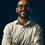# Why we turn the fraction upside down when dividing?

Hello everyone, I have a doubt.

Why a/b times c/d = a/b divided by d/c?

Why multiplying by the reciprocal is the same as dividing that fraction? I get the mechanics, I get that the division is the inverse of multiplication, but I can't really visualize it in my mind and I don't get WHY that is true.

Sorry if this question sounds dumb, but I really love the way we're learning math here at Brilliant, and I would love if someone could provide some visual aid or any guidance, really.Note by Davide Taraborrelli
3 months, 1 week ago

This discussion board is a place to discuss our Daily Challenges and the math and science related to those challenges. Explanations are more than just a solution — they should explain the steps and thinking strategies that you used to obtain the solution. Comments should further the discussion of math and science.

When posting on Brilliant:

• Use the emojis to react to an explanation, whether you're congratulating a job well done , or just really confused .
• Ask specific questions about the challenge or the steps in somebody's explanation. Well-posed questions can add a lot to the discussion, but posting "I don't understand!" doesn't help anyone.
• Try to contribute something new to the discussion, whether it is an extension, generalization or other idea related to the challenge.

MarkdownAppears as
*italics* or _italics_ italics
**bold** or __bold__ bold
- bulleted- list
• bulleted
• list
1. numbered2. list
1. numbered
2. list
Note: you must add a full line of space before and after lists for them to show up correctly
paragraph 1paragraph 2

paragraph 1

paragraph 2

[example link](https://brilliant.org)example link
> This is a quote
This is a quote
    # I indented these lines
# 4 spaces, and now they show
# up as a code block.

print "hello world"
# I indented these lines
# 4 spaces, and now they show
# up as a code block.

print "hello world"
MathAppears as
Remember to wrap math in $$ ... $$ or $ ... $ to ensure proper formatting.
2 \times 3 $2 \times 3$
2^{34} $2^{34}$
a_{i-1} $a_{i-1}$
\frac{2}{3} $\frac{2}{3}$
\sqrt{2} $\sqrt{2}$
\sum_{i=1}^3 $\sum_{i=1}^3$
\sin \theta $\sin \theta$
\boxed{123} $\boxed{123}$

Sort by:

Interesting thought! Unfortunately, it might not be something you can exactly visualize though. Think of it this way: When we do $10~\div~2$ for example, we visualize it by imagining that we have $10$ objects, and $2$ people who want to share it. That way, we physically split up the $10$ objects evenly into $2$ groups. But we if tried to do the same with $10~\div~\frac{1}{2}$, our visualization would fail, since it doesn't make sense to have $\frac{1}{2}$ a person, or $\frac{1}{2}$ groups of objects.

Problems like this arise with even simpler operations like subtraction. For quite some time, mathematicians would simply refuse to subtract a larger number from a smaller one, since doing this would give a number smaller than $0$, which to them was impossible! We of course today use negative numbers to get around this, but the concept still lacks a physical representation, since we can never have less than $0$ things.

Hope this helps! Maybe someone has a different suggestion...

- 3 months, 1 week ago

Hello everyone! Thank you tons for clarifying this idea for me and offering some proof. That indeed helped. I am still trying to wrap my head around those as I am still at the beginning of my math journey, but I do appreciate your help! Thanks!

- 3 months, 1 week ago

In China no one in middle school has this problem anymore. Maybe it is hard to visualise how multiplying by reciprocal is, but we can prove this: $a\div b=a\times 1\div b=a\times \frac{1}{b}. ~~~~~\text{ (Reciprocal)}$ $a\div\frac{b}{c}=a\div(b\div c)=\frac{a}{b} \div (1\div c)=\frac{a}{b}\times c=\frac{ac}{b}.$

- 3 months, 1 week ago

Hello @Jeff Giff! Thanks for this explanation. Can I ask you why a/b divided by 1 divided by c = a/b times c? Thanks!

- 3 months, 1 week ago

Oh! Umm... since a number’s reciprocal is one divided by it, $c$ and $\frac{1}{c}$ are reciprocals. So $\frac{1}{c}$ and $c$ are reciprocals of each other. Therefore $\frac{1}{\frac{1}{c}}=c.$

- 3 months, 1 week ago

Hi @Jeff Giff! Sorry for the delay I'm writing to you. I just wanted to let you know that I appreciated your explanation! Thank you

- 2 months, 3 weeks ago

$\blue{a}\div \red{b}=\blue{a}\times \red{b}^{\purple{-1}}$ $\Rightarrow \blue{\dfrac{a}{b}}\div \red{\dfrac{c}{d}}=\blue{\dfrac{a}{b}}\times \left(\red{\dfrac{c}{d}}\right)^{\purple{-1}}$ $=\blue{\dfrac{a}{b}}\times \red{\dfrac{d}{c}}=\dfrac{\blue{a}\times\red{d}}{\blue{b}\times\red{c}}$

- 3 months, 1 week ago

And negative numbers mean the opposite meaning!
I suppose nobody would use negative numbers like ‘I have -2 cakes’ or ‘he is -1.8 meters tall’. Instead, negative numbers are used in daily life to represent things of the opposite meaning, for example ‘I walked -1 meters south’ is equivalent to ‘I walked a meter north’.

- 3 months, 1 week ago

You're right, the only sensical way to think of negative numbers is as a direction, not as a quantity. But then we still have a slight problem, since now it would seem we've redefined what a "number" is. To most, it would seem that a number must be a quantity, since their original function was to count things.

- 3 months, 1 week ago

Finally, to visually represent a negative number, there’s always the number axis or a negative pointing vector maybe :)

- 3 months, 1 week ago